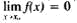# infinitesimal

(redirected from Infintesimal)
Also found in: Dictionary, Thesaurus.

## infinitesimal

1. Maths of, relating to, or involving a small change in the value of a variable that approaches zero as a limit
2. Maths an infinitesimal quantity
Collins Discovery Encyclopedia, 1st edition © HarperCollins Publishers 2005
The following article is from The Great Soviet Encyclopedia (1979). It might be outdated or ideologically biased.

## Infinitesimal

in mathematics, a variable quantity that approaches a limit equal to zero. In order that the concept of an infinitesimal may have an exact meaning, it is necessary to indicate the process of variation in which the given quantity becomes an infinitesimal. For example, the quantity y = 1/x is an infinitesimal for an argument x that approaches infinity, but for an x that approaches zero it proves to be infinitely large. If the limit of the variable y is finite and equal to a, then lim (y – a) = 0, and conversely. Thus, the concept of an infinitesimal can serve as the basis of a general definition of the limit of a variable. The theory of the infinitesimal is one of the methods of constructing a theory of limits.

In considering several variables involved in the same process of variation, the variables y and z are called equivalent if lim (z/y) = 1; if in this case y is infinitesimal, the y and z are called equivalent infinitesimals. The variable z is called infinitesimal with respect to y if z/y is infinitesimal. The latter fact is often written in the form z = o(y), which reads “z is o small with respect to y”. If y is infinitesimal here, then it is said that z is an infinitesimal of a higher order than y. Often, among several infinitesimals that take part in the same process of variation, one of them, let us say y, is assumed to be the principal, and the rest are compared with it. Then it is said that z is an infinitesimal of order k > 0 if lim (z/yk) exists and is not zero. If this limit is equal to zero, then z is called an infinitesimal of order higher than k. The study of the orders of different kinds of infinitesimals is one of the important problems of mathematical analysis. For the case in which the variable quantity is a function of the argument x, the following explicit definition of an infinitesimal results from the general definition of a limit: the function f(x), defined in the neighborhood of the point x0, is called an infinitesimal for x approaching x0 if, for any positive number ∊, there is a positive number δ such that for all xx0 which satisfy the condition ǀx - x0ǀ < δ, the inequality ǀf(x)ǀ < ∊ is satisfied. This fact is written in the form. In the study of the function f(x) near the point x0, one takes the increment of the independent variable Δx = x - x0 as the principal infinitesimal. The formula Δy = f(xox + ox), for example, expresses the fact that the increment Δy of a differentiable function coincides with its differential dy = f’(x0x with an accuracy within an infinitesimal of an order higher than the first.

The method of the infinitesimal, or (what is the same) the method of limits, is currently the primary method of substantiating mathematical analysis, which is why it is also called infinitesimal analysis. It replaced the exhaustive method of antiquity and the method of indivisibles. The method of the infinitesimal was outlined by I. Newton in 1666 and was universally accepted after the works of A. Cauchy. Using the infinitesimal, one can define such basic concepts of analysis as convergent series, the integral, the derivative, and the differential. In addition, the method of infinitesimals serves as one of the primary methods of applying mathematics to the problems of natural science. This is connected with the fact that most of the principles of mechanics and classical physics are expressed in the form of formulas relating infinitesimal increments of the quantities under investigation, and the infinitesimal is usually used to construct the differential equations of the problem.

S. B. STECHKIN